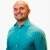# SAT Prep How to determine the perimeter of a triangle using similar trianglesThis lesson will look at an example problem involving perimeter. This lesson will give an explanation of how to determine the perimeter of a triangle using similar triangles with a step by step look to better... This lesson will look at an example problem involving perimeter. This lesson will give an explanation of how to determine the perimeter of a triangle using similar triangles with a step by step look to better explain the concept.
More... Collapse
16 Views